# Question-10 Suppose you take out a 30-year mortgage of \$100,000 with a fixed interest rate of 5 percent. You must make 360 equal monthly payments. Write an equation that defines what the payment is? (5

suppose you take out a 30- year mortgage of \$100,000 with a fixed interest rate of 5%. You must take 360  equal monthly payment write an equation that defines what the payment is?

This Homework Help Question: "Question-10 Suppose you take out a 30-year mortgage of \$100,000 with a fixed interest rate of 5 percent. You must make 360 equal monthly payments. Write an equation that defines what the payment is? (5" No answers yet.

We need 9 more requests to produce the answer to this homework help question. Share with your friends to get the answer faster!

1 /10 have requested the answer to this homework help question.

Once 10 people have made a request, the answer to this question will be available in 1-2 days.
All students who have requested the answer will be notified once they are available.

#### Earn Coin

Coins can be redeemed for fabulous gifts.

Similar Homework Help Questions
• ### You take out a 30-year fixed-rate mortgage for \$100,000 with an interest rate of 12% (APR). Part...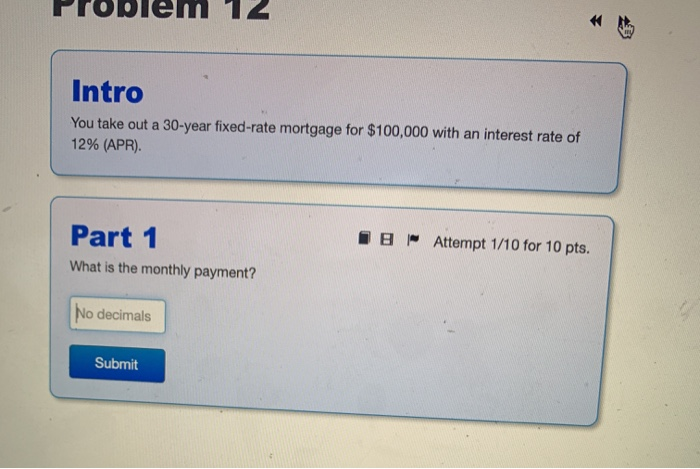You take out a 30-year fixed-rate mortgage for \$100,000 with an interest rate of 12% (APR). Part 1 What is the monthly payment?

• ### (1) What would be the monthly payment for the 30 year monthly mortgage (total 360 equal...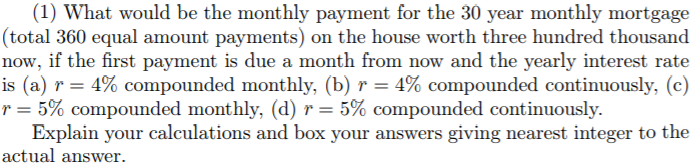(1) What would be the monthly payment for the 30 year monthly mortgage (total 360 equal amount payments) on the house worth three hundred thousand now, if the first payment is due a month from now and the yearly interest rate is (a) r-: 4% compounded monthly, (b) r-4% compounded continuously, (c) r-5% compounded monthly, (d) r--5% compounded continuously. Explain your calculations and box your answers giving nearest integer to the actual answer

• ### You plan to take out a​ 30-year fixed rate mortgage for ​\$ 200,000. Let​ P(r) be your monthly payment if the interest rate is​ r% per​ year, compounded monthly. Interpret the equations ​(a) ​P( 2) equ...

You plan to take out a​ 30-year fixed rate mortgage for ​\$ 200,000. Let​ P(r) be your monthly payment if the interest rate is​ r% per​ year, compounded monthly. Interpret the equations ​(a) ​P( 2) equals = 739.24 and​ (b) P'(2) equals = 100.01 . ​ (a) Interpret ​P (2​) equals = 739.24. Select the correct answer below. A. If the interest rate on the mortgage is 3​%, the monthly payment will be ​\$ 739.24 B. If the interest rate...

• ### Suppose that you are considering a conventional, fixed-rate 30-year mortgage loan for \$100,000. The lender quotes...

Suppose that you are considering a conventional, fixed-rate 30-year mortgage loan for \$100,000. The lender quotes an APR of 4.71%, compounded monthly; mortgage payments would be monthly, beginning one month after the closing on your home purchase. What would be your monthly mortgage payment?

• ### You plan to take out a 30-year fixed rate mortgage for \$225,000. Let PC) be your...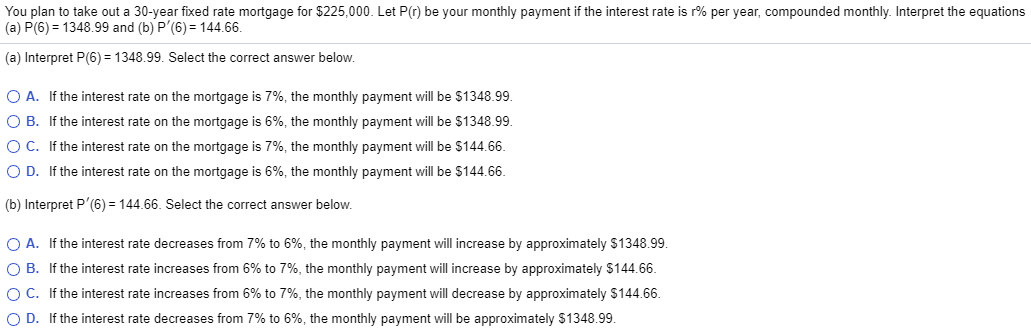You plan to take out a 30-year fixed rate mortgage for \$225,000. Let PC) be your monthly payment if the interest rate is r% per year, compounded monthly. Interpret the equations (a) P(6) = 1348.99 and (b)P (6) = 144.66. (a) Interpret P(6) = 1348.99. Select the correct answer below. O A. If the interest rate on the mortgage is 7%, the monthly payment will be \$1348.99. OB. If the interest rate on the mortgage is 6%, the monthly payment...

• ### Suppose you take out a 30-year mortgage for \$241119 at an annual interest rate of 6.1%....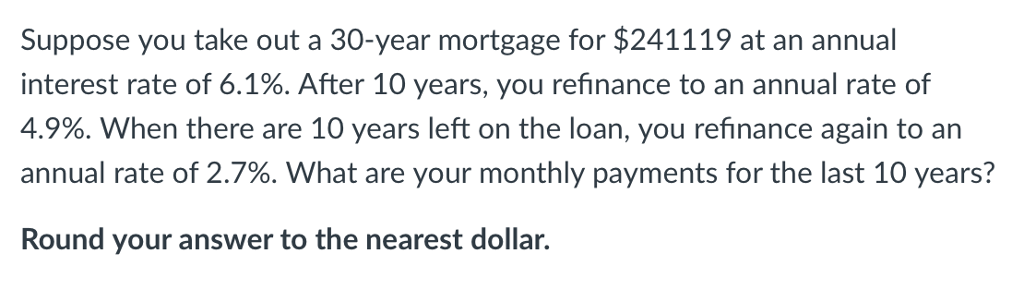Suppose you take out a 30-year mortgage for \$241119 at an annual interest rate of 6.1%. After 10 years, you refinance to an annual rate of 4.9%, when there are 10 years left on the loan, you refinance again to an annual rate of 2.7%. What are your monthly payments for the last 10 years? Round your answer to the nearest dollar.

• ### 11. Suppose that you take out a \$250,000 house mortgage from your local savings bank. The...11. Suppose that you take out a \$250,000 house mortgage from your local savings bank. The bank requires you to repay the mortgage in equal annual installments over the next 30 years. Suppose that the interest rate is 5% a year. Then what is the amount of mortgage payment each year? (a) \$16,263 (b) \$13,452 (c) \$12,583 (d) \$10,127 12. Consider a borrower that is approved for a standard 15-year, fully amortizing house mortgage with an original balance of \$500,000...

• ### suppose you took a \$100,000 15 year fixed-rate mortgage at 4.5% (APR) 3 years ago. Now...

suppose you took a \$100,000 15 year fixed-rate mortgage at 4.5% (APR) 3 years ago. Now the market interest rate has dropped to 4%, and you are considering refinance your mortgage. (1) What was the original monthly payment? (2) Suppose you just made the 36th monthly payments. What is the remaining mortgage balance? (3) If you refinance with mortgage with another bank and keep the remaining term (that is, 12 years until the mortgage is paid off), what would the...

• ### Ten years ago you obtained a 30-year mortgage for \$400,000 with a fixed interest rate of...

Ten years ago you obtained a 30-year mortgage for \$400,000 with a fixed interest rate of 3% APR compounded monthly. The mortgage is a standard fixed rate mortgage with equal monthly payments over the life of the loan. What are the monthly fixed mortgage payments on this mortgage (i.e., the minimum required monthly payments to pay down the mortgage in 30 years)? What is the remaining loan balance immediately after making the 120th monthly payment (i.e., 10 years after initially...

• ### 4. A. What would be your monthly mortgage payment if you pay for a \$250,000 home...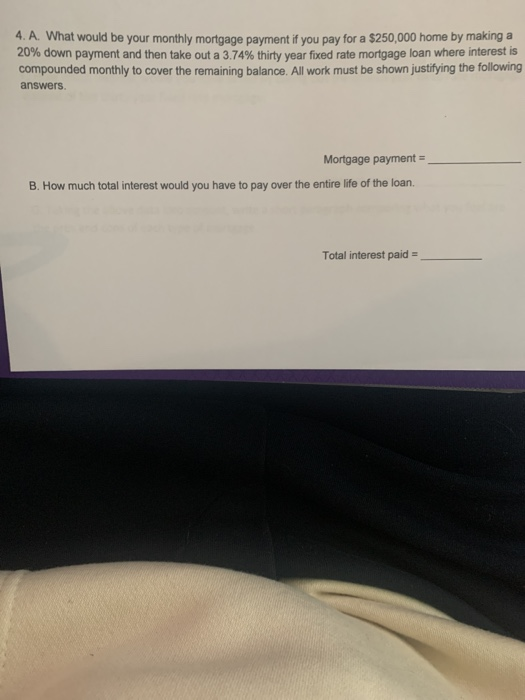4. A. What would be your monthly mortgage payment if you pay for a \$250,000 home by making a 20% down payment and then take out a 3.74% thirty year fixed rate mortgage loan where interest is compounded monthly to cover the remaining balance. All work must be shown justifying the following answers Mortgage payment = B. How much total interest would you have to pay over the entire life of the loan. Total interest paid = C. Suppose you...